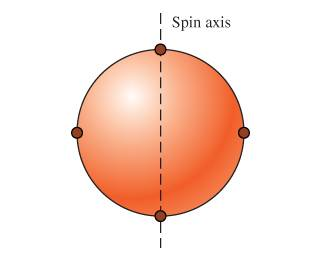# Calculating Friction Torque

## Homework Statement

A uniform spherical shell of mass 8.90kg with diameter 53.0cm has four small masses of mass 2.50kg attached to its outer surface and equally spaced around it. This combination is spinning about an axis running through the center of the sphere and two of the small masses .

This is the image to accompany the problemNOTE:

In this next problem two of the small masses have the axis of rotation running through them. Because their mass is very small compared to that of the sphere and they are so close to the axis of rotation, you can assume that their contribution to the moment of inertia is neglgible. You cannot assume that, though, for the masses that are far from the axis of rotation.

What friction torque is needed to reduce its angular speed from 80.0 rpm to 53.0 rpm in a time interval of 25.0 secs?

## The Attempt at a Solution

Mass of sphere = 8.90kg

Radius = 0.265m

Small spheres = 2.50kg each

First, I converted the rpms into rads/sec

80rpm = 8.377rad/s

53 rpm = 5.55rad/s

Then I found the angular acceleration

5.55rad/s-8.377rad/s/25 secs = -0.11308 rad/sec^2

I then calculated the moment of inertias for the big sphere and two small ones because you can neglect the others that the axis of rotation runs through.

I_BigSphere = 2/5MR^2

I_smallspheres=(2)MR^2

I_Big= 2/5(8.90)(.265m)^2

I_small=2(2.50)(.265m)^2

Add these two together = 0.601126

Then Torque = I$$\alpha$$

(0.601126)(-0.11308 rad/sec^2) = -0.0679 N*M?

## Answers and Replies

ideasrule
Homework Helper
Haven't checked your calculations, but your equations look good.

aw shucks, i just typed that in for the answer and it is incorrect.